# CR-manifold

(diff) ← Older revision | Latest revision (diff) | Newer revision → (diff)

In 1907, H. Poincaré wrote a seminal paper, [a6], in which he showed that two real hypersurfaces in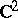are, in general, biholomorphically inequivalent (cf. Biholomorphic mapping; Hypersurface). Later, E. Cartan [a10], [a11] found all the invariants that distinguish one real hypersurface from another. The general solution for complex dimensions greater than two was given by S.S. Chern and J. Moser [a3] and N. Tanaka [a8], [a7].

The concept of a CR-manifold (CR-structure) has been defined having in mind the geometric structure induced on a real hypersurface of,.

Letbe a real differentiable manifold and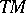the tangent bundle of. One says thatis a CR-manifold if there exists a complex subbundleof the complexified tangent bundle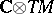satisfying the conditions:;is involutive, i.e., for any complex vector fieldsandinthe Lie bracket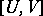is also in.

Alternatively, by using real vector bundles it can be proved (cf. [a1]) thatis a CR-manifold if and only if there exists an almost-complex distribution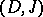on(i.e.,is a vector subbundle ofand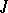is an almost-complex structure on) such thatlies in;for any real vector fields,in.

Thus the CR-structure onis determined either by the complex vector bundleor by the almost-complex distribution. The abbreviation CR refers to A.L. Cauchy and B. Riemann, because, forin,consists of the induced Cauchy–Riemann operators (cf. Cauchy–Riemann conditions).

A-function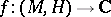is called a CR-function if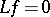for all complex vector fieldsin. A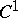-mapping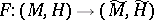is said to be a CR-mapping if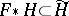, where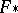is the tangent mapping of. In particular, ifis a diffeomorphism, one says thatis a pseudo-conformal mapping and thatand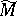are CR-diffeomorphic or, briefly, that they are equivalent. A CR-structure onis said to be realizable ifis equivalent to some real hypersurface of a complex Euclidean space.

Letbe the natural projection mapping. Then the Levi form foris the mapping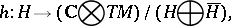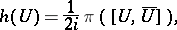for any complex vector fieldin. Ifis the real hypersurface ingiven by the equation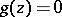, where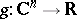is smooth, then the Levi form foris identified with the restriction of the complex Hessian ofto(cf. also Hessian matrix). Whenis positive- or negative-definite on, one says thatis strictly pseudo-convex.

The differential geometry of CR-manifolds (cf. [a4]) has potential applications to both partial differential equations (cf. [a2]) and mathematical physics (cf. [a5] and [a9]).

How to Cite This Entry:
CR-manifold. Encyclopedia of Mathematics. URL: http://encyclopediaofmath.org/index.php?title=CR-manifold&oldid=31185
This article was adapted from an original article by A. Bejancu (originator), which appeared in Encyclopedia of Mathematics - ISBN 1402006098. See original article